# SearchThisVideo: College Algebra – Lecture 1 – Numbers

#### SearchThisVideo

Watch video at 00:00
in this unit which I'm calling basics or
subtitled remembrance of things past
we'll look at some of the basic ideas
that are needed for the rest of the
college algebra course we'll look at
numbers which you can think of as the
raw materials for the course but they
are not what the course is about the
course is really about the operations
that you perform on numbers we'll look
at the language of mathematics a little
bit we'll look at exponents and exponent
notation we'll look at polynomial
expressions and we'll finish up with a
little bit of geometry so I hope you
enjoy it and here we are
the first part of basics we'll look at
our numbers and under numbers the first
thing we'll look at is an idea of
mathematics that will help us describe
numbers we'll talk about sets of objects
first of all so let's look at that sets
of objects now sets are what a
mathematician calls a bunch of things
now what will be in such a set or a
bunch or a collection numbers
expressions functions all these things
that we'll be talking about later in the
course but let me go ahead and give you
an example for example for example let's
look at the set of base 10 digits those
are the digits we use to write all of
our numbers in our number numeral system
and I'll give you the notation as we go
I'll use this curly brackets or braces
to start the set and then I'll list all
the digits inside with commas so we're
four five six seven eight and nine and
then end the brackets so these beginning
and ending items are called set brackets
sometimes they're called braces and they
are what indicate the beginning in the
end of the set all of the elements of
the set there's so few of them I can
simply list them all the elements are
separated musi by commas this is an
example of a finite set
it's a set with only a finite number of
elements in fact you can see that there
are exactly ten elements and what we've
done is we put it all together in one
place it's a collection of objects
that's all that a set is nothing nothing
fancy just a simple idea let's look at a
couple more examples here's an example
this should be familiar to anyone that
speaks English the set of 26 English

Watch video from 03:00 - 6:00

letters I will write that starting off
with the braces again and then list the
letters a B C and D and you know there
are so many that I don't want to list
them all so what I will do is I will put
dot dot dot in the middle and then a
comma and then I will list the last few
XY and Z these dots sometimes referred
to as an ellipsis is a way of indicating
that the set continues on and in this
case I happen to know the ending so I
cannot put on the the last few elements
this again is another finite set now as
you might expect in mathematics there
are lots and lots of sets that are not
finite we call those infinite sets so
let me give you an example of one of
those and yet again show you this
notation example here is another set
this is the set of positive even numbers
now as we go on we'll talk about numbers
in detail but I think you probably
remember what these are numbers like 2 4
6 8 10 etc now you see I put the dots at
the end and I put nothing afterwards I
don't know how it ends because there's
an infinite number of even numbers
there's always more beyond wherever I
stop so this is a perfect example of an
infinite set and this is one way of
writing down such a set we will learn
other ways as we go but I wanted to show
you both finite and an infinite set now
there's one more idea that we want to
touch on before we start talking about
numbers
and here I'll show you in an example
what I mean here is a small set the set
two four six
there's a set with only three elements
in it this is what's called this is
what's called a subset of that larger
set we saw on the other page 2 4 6 8 10
etc the infinite set of real even
numbers you see that these three
elements are contained in this set this
is a smaller set this is a sub set of
the larger set so if you were to look at
this in a picture say if this shape
describes the set then what we have here
is a small part of that set called a sub
set now I will use that terminology
throughout the course and if we need to
say anything more about sets and sets of
objects we'll introduce that terminology
as we go but now let's go back to the
list and see what kind of numbers we
will first look at will first look at
natural numbers these are sometimes
called the counting numbers what are
they natural numbers these are numbers
that we really take for granted these

Watch video from 06:00 - 9:00

are numbers that we didn't have to
invent as we have had to event other
numbers these numbers can be thought of
as just existing they are often denoted
the set is often denoted by a boldface N
and that's the way I'll write that and
what is this set well its consists of
these numbers 1 2 3 4 etc and then
sometimes we do this also we'll put an N
here to indicate the variable that I
will use or the letter I will use to
stand for such numbers and then a comma
and continue on out to infinity so this
is a commonly used variable for natural
numbers and I'll use it from time to
time these will be taken as given these
are not invented there's nothing to
describe these are simply the starting
place for the number systems we will
look at but you know there are lots of
numbers within this set of numbers that
are quite that are that are quite
interesting so let's go ahead and talk
because they will have bearing later
there's a set of numbers that are called
prime numbers now why would I want to
talk about those well as in chemistry it
is helpful to look at what you might
consider the atoms of the material that
you are discussing prime numbers can be
considered the atoms of the natural
numbers for example let me go ahead and
define what a prime number is P is prime
call it a prime number if well first of
all P is greater than 1 because 1 is not
considered a prime number and what makes
a prime number and atom P is divisible
only by two things only by 1 which
divides into every number and itself P
what do these numbers look like well I'm
sure you've seen these in your life the
set of prime numbers looks like this the
first one is 2 which happens to be the
only even prime number then 3 5 7 not 9
because remember 9 would be 3 times 3 11
13 17 etc there are an infinite number
of primes and this is what they look
like numbers like this
they're only divisible they can only be
factored words that I will be describing
soon into themselves times 1 so those
are prime numbers now with prime numbers
since we can think of them as the atoms
of the natural numbers you might expect
that we ought to be able to write all
natural numbers in terms of prime
numbers and so we can and this is such a
famous result I just want to go ahead
and introduce it to you it's called the
fundamental theorem fundamental theorem

Watch video from 09:00 - 12:00

of arithmetic arithmetic you may ask why
are we studying arithmetic in an algebra
course
as you'll see it really is going to be
the basis for much of what we do and
it's probably a theorem that you haven't
seen in this form but I think you know
what it's going to say any natural
number that is to say any of those
counting numbers we saw before any
natural number can be factored now I
haven't officially defined the word
factor but a factor is simply a thing
that is multiplied so to factor a number
like 12 would be to write it as a
product of two other numbers it can be
factored uniquely there's only one way
you can factor it and that one way is
except for the order of the factors
except for the order of the factors
because as you may remember from your
knowledge of the numbers that numbers
like two times three are the same thing
as numbers three times two into a
product of prime powers prime numbers
prime powers so to read to say this one
more time any natural number can be
factored uniquely except for the order
of the factors into a product of prime
powers now let me give you some examples
so you'll know what it is I'm saying
here and once I do I think you'll see
that this is familiar let me take the
number I mentioned a moment ago twelve
twelve as you know can be written as
three times four that may be the first
thing that comes to mind but four can be
written as two squared so this can be
written as 2 squared times 3 now 2 is a
prime number to a power this is two
times two that's what the two up there
means and 3 is another prime number let
me do a few more examples 70 can be
written as 2 times 5 times 7
for example 111 can be written as 3
times 37 etc all the natural numbers can
be written as products of prime numbers
or possibly powers of prime numbers
and as I said before and we'll define it
again in a little bit factors are things
that are multiplied now before I leave
this topic I don't want to mislead you
generally factoring numbers is hard if
you try to factor it is very hard to do
in fact that's being used in security
systems these days for that very reason
but the kind of factoring we will do is
fairly simple and so I'll pause now and

Watch video from 12:00 - 15:00

give you a chance to remind yourself of
a few things now we'll continue our
development of the numbers by looking at
integers so what are the integers the
integers is a larger set than the
natural numbers the integers are often
denoted by a capital Z you may say what
does that have to do with integers
there's no Z in the word integer well
just for the historically minded among
you this comes from the German words all
N which stands for numbers so that's
what the symbolism comes from and what
are the integers how do they differ from
the natural numbers well the way I'm
going to define the integers is to give
you a sense of why one would need the
numbers so let's let imagine that we're
moving ahead in the course here and we
want to solve a certain very simple
equation suppose we want to solve the
equation X plus 1 equals 1 we want to
find a number X that will make this
equation true well you know from your
own experience that there is only one
number that will make this true we need
what we need the number 0 but in the
natural numbers there is no 0 so 0 has
to be added to that and that will become
one of the integers let me show you one
other small equation to suggest why we
need another set of numbers suppose we
want to solve X plus 1 equals 0
using that new number zero now from what
you know what would you have to add to
one to get zero well you'd have to add
we need a number like minus one but
again the natural numbers don't have any
negative numbers in them so we have to
all in we're going to get a set that is
the integers here is that set the set Z
and it will be infinite in both
directions so I'll have to start off
with dots and then I'll give you a few
of the numbers minus 4 minus 3 minus 2
minus 1 then there's that special number
0 so I'll put it on its own line alone
and then we'll continue 1 2 3 4 etc out
to infinity now there's a more compact
way to write this so I'll show you that
if we put 0 at the front and then we put
a plus and a minus sign here for 1 so we
can imagine plus 1 and minus 1 being
next to each other and then plus or
minus 2 plus or minus 3 plus or minus 4
etc out to infinity now this is just
more compact so this is a more compact
way of writing this set but we have now
expanded our set of numbers we no longer

Watch video from 15:00 - 18:00

have simply the natural numbers notice
that the natural numbers are right here
they're included in the integers we have
negative numbers and a reasonable way of
describing the reason for that is to say
that we needed to solve certain kinds of
equations so let me show you a couple of
things in an example about these numbers
these are things that you probably have
seen much of your life I'll just remind
you of a couple of things for example if
I take 0 times 5 and notice I've already
started introducing a notation that you
may not be familiar with I'm using a dot
for multiplication again I will talk
about that a little bit later on but you
can see that I'm multiplying 0 times 5
well you know what happens when you
multiply a number times 0 you get 0 so
there's a fact from your past I hope and
if we take 0 times
82 we're going to get zero it doesn't
matter what number you choose zero times
that number is zero what else can we say
how about zero plus a number like zero
plus seven
well that is seven the zero contributes
nothing so you know that adding zero to
any number will result in the original
number coming back how about negative
numbers what are some of the properties
you probably have seen before well
suppose we take two numbers like minus 5
and minus 2 and multiply them together
well you'll get 10 in fact to get plus
10 because a negative times a negative
is a positive
minus 5 plus 2 well as you know 2 minus
5 will give me a minus 3 etc so the
kinds of facts that you you should be
familiar with are the facts about the
elementary integers and the properties
they have so let's go ahead and look at
another set of numbers that is built
from integers so we'll go back to my
list and after integers we will look at
rational numbers this is where we take
integers and we create a new set of
numbers
and I'll show you exactly how that's
done rational numbers now rational
numbers have their own bold-faced letter
symbol Q and this time the Q stands for
quotient so that seems to make good
sense what does a rational number look
like well then what you previously
called in grammar school fractions P
over Q that is a rational number what
are the P and Q the P and the Q are
integers so they come from that set Z
that we saw a moment ago and we also say
that Q is not 0
now we're forbidding the bottom to be 0
the bottom of the fraction is not 0 and
I'll explain why in a little bit but
we're going to say the division by 0 is
not allowed it is undefined there's a

Watch video from 18:00 - 21:00

good reason for that but this of course
is what you call before as
set a fraction you might also have used
the word ratio which is where rational
comes from in the description of these
and now let's look at a few examples
just to remind you what we're talking
about here examples you know what these
are 1/2 2/3 1113 574 over 201 etc those
are all examples of rational numbers
they're fractions
some of them as in the case of the last
one are improper fractions the top is
bigger than the bottom but there's still
rational numbers now let me note that
once again we have increased our number
system without losing anything all n is
in queue in fact all Z the integers all
of that set is in queue how do I know
that well
since if you take a number n from either
Z or the natural numbers and you put it
over 1 lo and behold you have a rational
number
but of course n over 1 is still N and so
these numbers from here can be
re-written as n over 1 and they appear
as rational numbers and for example 8
over 1 of course is 8 and so on so
that's what rational numbers look like
now I said a moment ago that division by
zero is not allowed in the natural in
the in the rational numbers so let me go
ahead and give you that warning and give
you a reason why that is true so that we
don't have any mysteries here warning
division by zero is what's called
undefined
now you might say well it's undefined
why doesn't somebody define it well as a
reason it's undefined because you cannot
define it as a number and end up without
question rather is why why is it
undefined here's why let us suppose
let's take a simple case because if it
fails here it's probably going to fail
for the rest suppose 1 over 0 and I'll
put it in quotes suppose 1 over 0 is
taken to be a number well if one over 0
is taken to be a number it must share
all the properties that the other
numbers we've seen satisfy that is going
to lead us to a problem watch then
consider the following situation and
this is just one of many possible
situations I could describe consider the
following 0 times this news purported
number 1 over 0 now I can make two

Watch video from 21:00 - 24:00

different arguments on the one hand I
can say this must be 0
why here's my reason since as we saw a
moment ago 0 times x equals 0 for any
number that we've seen X you multiply a
number by 0 it is 0 that's what I've
done here multiplied this purported
number by 0 so I claim it's 0 fine on
the other hand I can also make the
following argument that this is equal to
1 and what's my reason there since
copying what I have here if you have x
times 1 over X that's the same as x over
X and of course that's 1 for any X well
if I assume that you can divide by zero
and that one over zero is a legitimate
number then I end up with it
being multiplied by zero I get either
zero or one I can't have both so there
is no way no matter what I define this
to be I will end up with a problem like
this that's a contradiction so one over
zero can't be defined because if you
define it you're going to have
contradictions arise and you don't want
that in your number system so in case
anyone was wondering why division by
zero is not allowed there's the reason
alright let's look at some operations on
rational number rational numbers and
I'll say some operations on Q and these
are fraction operations these are the
kind of things you've had experience
with over the course of your elementary
school career but now we'll put them in
a form that's more algebraic for example
suppose you have the fraction a over B
times and other fractions C over D what
does that simplify to how do you
multiply two fractions well this is the
easiest of the operations this one's
easy
you multiply the top a C and over the
bottom B D now there are a couple of
things going on here with notation that
we'll have to discuss later I'm using
the dot here again for multiplication
and also over here I'm using nothing I'm
just putting the numbers next to each
other for multiplication so I have AC
what if I took the fraction or rational
number a over B and I wanted to divide
it by the fraction or rational numbers C
over D how is that done well this is how
it's done you just copy over the top one
a over B and then we're going to
multiply it times the bottom one but
we're going to change the bottom one to
what's called its reciprocal now that's
a fancy older word it just means flip it
over

Watch video from 24:00 - 27:00

so if it's C over D here we'll multiply
it by D over C and then of course this
looks just like with the case above so
this becomes ad over BC and that's how
you divide two rational numbers the top
one just stays the bottom one comes over
multiplies and gets flipped over so that
should be something that you've seen
before and we've covered two operations
now multiplication and division there
are two more there's the addition and
subtraction so let's just go ahead for
the sake of completeness look at let's
look at a over B plus or minus C over D
now of all of the operations this is the
hardest these are the hardest because
but the reason is that a over B and C
over D are divisions in themselves and
pluses and minuses are different kind of
operation so we have to decide how we're
going to do this and the way that this
is done is we get what's called a common
denominator we make the fractions have
the same number at the bottom and the
easiest way to do this is to take a / b
multiply it by whatever i need on the
bottom and i'm going to take the product
of these two numbers as my new
denominator so I need a d down there and
since I don't want to change anything
I'll multiply a over B times D over D
remember D over D is 1 so I don't change
anything then plus or minus C over D I
do the same thing except I multiply by B
over B the advantage is now the bottom
is BD and the bottom is BD here so I
will get a new fraction with the
denominator or a bottom BD and the top
will be ad plus or minus BC and that is
how you add or subtract two fractions
this number at the bottom here by the
way is often called a common denominator
there's one of those older words
denominator we'll just call it bottom
for the most part and likewise we'll
refer to the tops of the fractions
instead of numerators we'll call them
top most of the time
alright let me go ahead and do a few
numerical examples just to give you a
chance to warm up a little bit
example if I take two fifths and I
divide it by 1/7 remembering the process
I copy down two fifths and then I
multiply it by this fraction flipped
over that's seven over one and so I will
have fourteen fifths and so I've divided
two fractions and ended up with another
fraction similarly I can do a simple
addition maybe take 1/3 plus 1/5 I take
the 1/3 I multiply the top and bottom by
5 over 5 plus one-fifth multiply top and
bottom by 3 so my denominator will be 3
times 5 or 15 in both cases have a new
fraction the bottom is 15 the top is 5

Watch video from 27:00 - 30:00

plus 3 so I end up with the fraction 8
15 now you'll have a chance in a little
bit to practice some of this but these
should be simple operations I hope
another note I want to make before we
have a chance to stop note in this
example I will illustrate another idea
suppose you're faced with the fraction 4
sixths well that is more complicated
than it needs to be if you recognize
that there's a factor common to the top
and the bottom you can then remove it as
follows if you realize the top is 2
times 2 and the bottom is 3 times 2 and
of course here's where factoring comes
in if you can do it you see that what
you've got is a new fraction here 2 over
2 which is just 1 so this can simply be
rewritten as 2 over 3 this is nice
because now 2 & 3 have no factors in
common they are in fact prime numbers
and that's a much nicer fraction than
the original and when you do this
this whole process is called put into
what's referred to as lowest terms
put this into lowest terms and that just
means you find out if they have common
factors the top and the bottom and you
rewrite them and those common factors
it's called cancelling but of course you
realize is just rewriting 2 over 2 as
the number 1 all right one final note
for rational numbers before we move on
to the next set of numbers my final note
here on rationals is do not here I'm
going to give you a stricture
do not use mixed numbers or mixed number
notation this is a notation that's very
common in the elementary schools like
for example 3 and 1/4 and I'll tell you
why because 3 and 1/4 means 3 plus 1/4
that's what you mean when you write down
3 and 1/4 you mean 3 plus 1/4 but now
when we write numbers next to each other
we're assuming for the most part
multiplication so we don't want to use
this notation and so I highly recommend
that you do not do that it's an older
notation and I would suggest that you
simply ignore it and use another form
all right let's go back to my list and
look at one last set of numbers before
we take a pause the last set of numbers
are the irrational numbers so let me
write that down
the irrational numbers Irrational's

Watch video from 30:00 - 33:00

Irrational's these are ones that are not
rational and let me again give you a
reason by presenting you with a simple
equation that will require an irrational
to be solved if you want to solve very
simple equation like X to the power 2
equals 2 so I'm looking for a number
here that when multiplied by itself
that's what the 2 means I get 2 but
there are no natural numbers lower than
2 that will have that property the only
natural number
two is one and one times one is one so
if I'm going to find a number it's
outside of my system what do we need
well you remain may remember the
notation that's used for this we need
either plus or minus what's called the
square root of two and you may notice
the way I write this with a little tail
I'll remind you of that later but this
is a number that one squared gives me -
this number is not an integer it's not a
natural number it's not a rational
number it is a new number we must add it
to our set Q which was the last set of
numbers we were looking at what other
kinds of numbers might not exist in two
well the set of irrational is rather
large and it's a mixed bag it's really a
motley bunch of numbers square root of 2
square root of 3 another famous number
you may know PI another number from that
rises in calculus II these are all
examples of irrational numbers they are
oddballs if you like they are not
defined as quotients of other numbers
like rational numbers in fact they're
not defined in a direct manner at all
they're simply all the numbers that are
left over when you picked out the
rational numbers there are a lot of them
in fact let me just give you one little
fact here in fact there are surprisingly
there are more Irrational's
then rationals these very odd strange
numbers that don't seem to fit any
pattern here there are in fact more like
that than there are rational numbers now
I won't go into that here but on that
note we'll pause and you can explore the
number systems we've already looked at
now as you've had a little practice with
rational numbers and the other numbers
we looked at earlier will now put them
together with the Irrational's and
produce a set called the real numbers so
let's examine that the real numbers also

Watch video from 33:00 - 36:00

have a symbol that's very common they
will be often denoted by a capital R
bold-faced R oh and one thing while I'm
here real numbers these numbers are no
more or less real in the colloquial
sense than any other number all numbers
are equally real this is simply a
technical term we put that up here this
is a technical term that we use to
describe the set I'm about to describe
so there's nothing more real about these
than any other numbers all right what is
the set of real numbers well it is the
set that contains Q and Q remember the
rational numbers and all the
Irrational's
so you take all the rationals and all
the Irrational's together and that forms
what we refer to as the set of real
numbers now this is the primary set that
we are going to be looking at in college
algebra this is the set we'll spend most
of our time in now let's go back to my
list because there's a way of talking
about these numbers that is quite nice
it takes advantage of geometry the real
line from numbers to points so we're
going to take numbers which are in the
realm of arithmetic and algebra and
connect them up with a geometric idea
this will be called the real line
so here's what we're doing give you the
overall view we're taking a geometric
object points alright
points which lie on a line and we are
going to put them in what's called
one-to-one correspondence with the real
numbers and real numbers of course are
an arithmetic or algebraic object so
we're connecting up algebra and geometry
here and it'll be very fruitful when we
have them connected to be easy to see
one idea from the other so let's go
ahead and write down what the real
number line looks like it is of course a
line so here's a line and I'll put
little arrows at the end so I indicate
that this goes on forever in both
directions now as it stands now it's a
geometric object with points what I'm
going to do now is start labeling the
points so that it gets algebraic
notation connected with it so the real
numbers become labels for the points on
this line first of all I'm going to
label what you might consider the center
of the real number system the number 0
the numbers go to the right and to the
left from there the rightward direction
gives you positive numbers the leftward
direction gives you negative numbers now
0 I will place anywhere so I'll even
mark that down anywhere let me also
point out that this is also referred to
as the origin so you can think of it
also as the origin the place where all
the numbers sort of begin then
arbitrarily I will pick a distance say
to the right and I will mark that as my
1 unit distance so this is an arbitrary
arbitrary 1 unit I just make a choice

Watch video from 36:00 - 39:00

but once I have that I can now put all
the other real numbers on here without
any difficulty using that as my
measuring device I can say then that 2
will be there 3 will be there four will
be there and so on in the other
direction - 1 - 2 - 3 and so on and you
might say well where are all the other
numbers where are say the rational
numbers well we just need to label them
between 0 & 1 at the halfway mark is
probably a good place to put the number
1/2 if we went a little further B
one we might find a place for oh the
number ten ninth say and where would
square root of two be well square root
of two as it turns out would appear in
square root of two the Irrational's also
live on this line what about in the
negative direction well down here
somewhere maybe is the number minus
thirteen 11s and so on all the numbers
we've seen the rationals the natural
numbers the integers and the
Irrational's can be considered lying
along this line and two just to
emphasize what I said about direction
let me write two large arrows here there
is one going to the right here's another
one going to the left the one to the
right I'll write right in there and
right means what it means increasing so
as you move toward the right no matter
where you start even if you start over
here you move from minus 3 to minus 2
you've gained by 1 as you move to the
right you gain as you move to the left
that is decreasing the numbers go down
as you go to the left so if you move
from 2 to 1 it's gone down but if you've
removed from minus 1 to minus 2 you've
also gone down by 1 so you have left and
right alright let's go back to my list
and we'll talk about another aspect of
the real numbers we looked at real
numbers and the real line from numbers
to points now we'll look at the fact
that real numbers are ordered and you
can see that from the line but we can
say even more so our is ordered that
means that some numbers are bigger than
other numbers it's a very commonsensical
idea and as I said before see the line I
guess I could stop there and say that's
all there is to it but I want to
introduce a notation so let me show you
something we can take the real numbers
and we can write them a new way we can
write them in this way we can say that
they are the set of all the positive
numbers
now that includes all the numbers we've
seen that happened to be positive zero
that dividing number and then all the
negative numbers now those are three
sets that we've joined together to make

Watch video from 39:00 - 42:00

the real numbers and the reason I
brought this up is for the following
definition suppose we have two numbers a
and B and suppose they're in the real
numbers so we have two numbers in the
real numbers we are going to say the
following we're going to talk about
which one's bigger we will say that a is
greater than B if a minus B is positive
now think about what that means a minus
B is positive only if a is bigger than B
when you take away B you have something
left over well that's what we want this
to say we want this to mean bigger the
way the notation works is you have this
triangle shape angled shaped object the
big side the open side is where the big
number goes and the point the small side
is where the small number goes let's go
ahead and continue this a equals B if a
minus B is well if they're equal and you
subtract one from the other
you should get zero and so you do and
finally a is less than B if and this
time B is the bigger one so where will a
minus B be if B is the biggest one
bigger one and you subtract it from a
you're going to get a negative result so
here is the way you can describe numbers
one of these three properties will
always hold when you have any two
numbers out of the real numbers in other
words we have order
the real numbers have order any two
numbers one is bigger than the other or
they're equal
all right well with that in mind let's
extend that notation a bit likewise
there's another notation we like to have
we will define a greater than or equal
to B and you heard me say it and that's
what it is defined to be this means a is
greater than B or possibly a is equal to
B and we put it together in a single
nice compact notation we also can define
a is less than or equal to B in the same
way this means that a is less than B or
that a is equal to B so this allows us a
little flexibility alright it's time to
look at some examples here so in fact
let's go to a new page so we have plenty
of room here's an example X greater than
4 now this is an algebraic statement

Watch video from 42:00 - 45:00

about members I want to write it using
the real number line so I could turn it
into a geometric statement well here's
my real number line here say is 0 let me
mark off 4 because that's the number I'm
interested in and I want all to
represent all the numbers X that are
bigger than 4 now this doesn't include 4
so the way I will do that is I'll put an
open dot at 4 that indicates there's no
point involved and then I'll mark off
all the points going to the right with a
slightly thicker line and an arrow at
the end so this inequality means this
picture this is geometry folks this is
algebra by connecting the two it becomes
easier to see what this means it means
all the numbers right above 4 and going
off to the right forever
let me give you another example it's
very important that you visualize these
things example here's another one that's
more complicated minus 1 is less than X
is less than or equal to 3 now we
haven't seen this before let me go ahead
and explain that this means what does
this mean this means minus 1 is less
than X that's the left-hand part and
simultaneously at the same time X is
less than or equal to 3 that's the
second part so both of these things must
hold at the same time now it's very easy
to draw a picture that illustrates this
in fact it's easier to draw the picture
than it is to look at the double
inequality here so the two numbers
involved are minus 1 and 3 that's all
I'm going to mark minus 1 and 3 I don't
care about 0 it's not part of what I'm
looking at this time now I want my X's
to be greater than minus 1 and not
include minus 1 so I'll put an open dot
at minus 1 on the other direction I want
X to be less than or equal to 3 but I do
want 3 to be included so I'll put a
closed dot there so I'll shade that in
and what am I looking for all the
numbers that are in between here so I'll
try and shade this all in so it looks a
little thicker and now this inequality
up here is this geometric set so
algebraic idea geometric idea that's
what you want to try for when you're
trying to understand this all right
there is a warning I want to give you
Oh morning and this is very common error
so I want to make sure that you don't do
this the statement that looks like this
the statement that now put it in quotes
say X is less than minus 2 or X is

Watch video from 45:00 - 48:00

greater than or equal to 5 okay which
means let me go ahead and show you the
understand what this means which means
what well it means let's see there's the
line there's minus 2 and there is 5
because -2 and 5 of the two numbers I'm
interested in less than minus 2 means
that we do not include minus 2 but we
can go less than that and any number
less than that which be all of these X
greater than or equal to 5 we do include
5 and we go to the right any number
that's greater than that is allowed so
this set is a set in two pieces okay
this this statement I'm sorry I forgot
to put the end of my quotes there the
statement X less than minus 2 or X
greater than or equal to 5 which means
this picture cannot cannot what cannot
be collapsed or if you like the word
re-written as and let me go ahead and
bring this back so you see what I'm
about to do I have X is less than minus
2 so you might want to think of X as
being in the middle like it was before
in the previous example X is greater
than or equal to 5 it cannot be
rewritten this way X less than minus 2
and greater than or equal to 5 because
minute you'll see that that's impossible
you're asking for numbers X which are
simultaneously greater than or equal to
5 so numbers that are bigger than 5 or
possibly equal to 5 and at the same time
less than minus 2 so it would have to be
a number that's both positive and
negative which can't happen this is
nonsense
so when you see statements as in this
earlier example X is greater than minus
1 less than or equal to 3 that has to be
very carefully qualified you have to
realize that there are numbers in there
and then draw a picture if you draw the
picture it's very difficult to make that
error but I wanted to warn you against
that all right before we leave this
topic of order sometimes we like to talk
about a group of real numbers that are
all together on a real on the real line
and they are referred to as intervals so
intervals on the real line and let me go
ahead and show you how we're going to
notate this notation open ends you've
seen from the previous examples of
sometimes we don't want to include the
number at one end of an interval open
ends where say endpoints are not

Watch video from 48:00 - 51:00

included as you saw in the previous
examples we will use either an open
parenthesis on the left or an open
parenthesis on the right similarly for
closed ends now these are endpoints that
will include the point at the end this
is where endpoints are included we will
use another notation square brackets or
square brackets going the other way so
these are the common accepted standards
for this kind of notation now let me
describe the intervals what is an
interval I said it was a set of real
numbers there are in fact going to be
nine types of intervals
depending on what part of the real
number line we choose nine types the
first group there will be two groups the
first group will be a bounded group and
you'll see what I mean for example the
first type of interval will be called an
open interval it will look like this a
comma B will write it exactly that way
what will that mean look at the picture
and you'll see why the notation was
invented if this is the real number line
and that those are the points a and B
now I do not want to include a I do not
want to include B but I do want all of
the points that are in between the two
in here that is what this symbol in the
middle represents the parentheses
indicate that a is not included B is not
included and a and B are simply the
start and the end and all the numbers in
between are indicated by this open
interval let's go ahead and look at what
are called half open or I guess if
you're pessimistic half closed intervals
so you can call them whatever you like
they will have one end open and when in
close so suppose a is the open end B is
the closed end or the other way around
a is the closed end B is the open end
now I can draw pictures for both of
these also mark off a and B a and B now
in this first one I do not want to
include a so I'll put an open hole at a
I do want to include B see that's what
the square brackets are includes B so
I'll shade in B and then the interval is
all the numbers in between and in this
case including the point at the right
endpoint if I look at this half open or
half closed interval this time I want to
include a so I'll close a shade in that
point but I do not want to include B so
I'll put an open hole there and then

Watch video from 51:00 - 54:00

I'll just shade in everything in between
more or less and now I have an interval
where I include the left
end point and finally as you might
expect if we have open and half open or
half closed we finally should have
closed and that would be square brackets
on both ends and you're probably ahead
of me on this one if this is a and this
is B we want to include both a and B and
we shade in between and we now have an
interval that includes both of the
endpoints the left and the right
endpoint and all of these intervals here
all the ones you see here are bounded
they don't go beyond a or B in any one
of these cases
that's what bounded means they have
boundaries on both ends now let's look
at the other type because I've only
shown you four types here there are
another five that I claimed exist these
will come under the category of
unbounded intervals so these are
unbounded but before we talk about
unbounded if we are going to have an
interval that goes off in one direction
forever or in the other direction
forever
I need a notation for that I don't have
a notation yet so let's invent one we
either going to go all the way off to
the right or all the way off sorry all
the way off to the left or all the way
off to the right well here's the
notation that's used for this to say go
all the way off to the left is to say go
to minus infinity to the right it's to
go to infinity that can also be written
plus infinity if you like now before we
go any further in there any
misconceptions these are not real
numbers
these are not real numbers what they are
are just handy symbols they're very
handy they will allow us to say go off
to the left without stopping or go off
to the right without stopping but they
are not real numbers what that means is
you can't add subtract multiply or
divide them or do anything that you do
with real numbers so now I'm ready to
write down the last five intervals I
promised you so we can have an interval
that includes the left endpoint but goes
off to the right forever you might
notice that I've put in open parentheses
on the right side that makes sense
because I can't have a closed
parenthesis there that would mean I
include the last number but there is no
last number so I have to put an open end
whenever there's an infinity around
what would the picture look like for
that well if this is a I am including a
this time and now I'm going off to the
right without stopping unbounded
similarly I can start a on the Left

Watch video from 54:00 - 57:00

without including a so I'll have a
picture where it's an open circle and
then I go off to the right without
stopping and then similarly I can go in
the other direction minus infinity
indicates go to the left without
stopping B can be included or minus
infinity B can be not included and these
as you might expect will look something
like this draw two lines there there's B
in both cases the first one I include B
and go to the left in this case I do not
include B and go to the left now that's
eight of the nine integrals in intervals
rather what is the last one well the
last one is everything nobody usually
writes this as the last one but let me
indicate it minus infinity to infinity
what would this look like well that's it
everything all of our
now if you want to write all of our
usually you're right simply are you
don't write the interval but it's nice
to know that it can be included in this
notation that we've got so having this
new notation we can rewrite what we did
in previous examples so previous
examples just to show you how this can
be used can now be re-written as the
following well this is a nice way to see
that this notation is compact one of the
examples I talked about X greater than
four this means that X is in the set the
interval from 4 to infinity greater than
4 means I do not include 4 that's why I
have the open parenthesis on the left
and greater than 4 doesn't have any
boundary so I go off to infinity here's
another one from before I had minus 1
less than X less than or equal to 3 what
is that going to mean that means X is in
the interval open minus 1 because I'm
not including minus 1 and on the other
end including 3 so this is a half open
or half closed interval including 3 and
my final example X less than minus 2 or
remember X greater than or equal to 5
that means that X is in the first one X
is less than minus 2 that's going to be
in going from minus infinity to minus 2
open or because X can't be in both at
the same time remember X is greater than
or equal to 5 X in the interval starting
and including 5 so it's closed bracket
square brackets going off to infinity to
the right so there I've rewritten the
answers to those previous problems in
this nice compact interval notation

Watch video from 57:00 - 60:00

which you will see for the rest of the
course so with that let's pause for a
moment so you can practice some of these
real number facts
now let's continue our exploration to
the real numbers by looking at the real
line distance between points first of
all we're going to make it very simple
we're going to talk about the distance
from zero one particular fixed point on
the real number line so let me show you
what I mean
here is the real number line and here
somewhere is 0 which is the origin if
you recall now suppose we have a number
on the line say I'll write it to the
right the number a now it has a certain
distance from zero we're going to give
that distance a name but before I do
give it that name I want to point out
that there's another number that is the
same distance from zero as a is if we
just go to the left of zero to right
about there the same distance a we come
up to the number minus a now minus a
also is the same distance away from zero
so both of these numbers are the same
distance away so with that in mind we're
going to define what that distance is
definition see before we talk about the
distance between two arbitrary points
we're going to take the distance between
the number and arbitrary number and a
fixed point the zero point and here it
is the distance from a real number X
okay we'll just call it X this time for
the time being to 0 which is the origin
is called now this is a name that
probably could be better but will give
you the name this is called the absolute
value of x
now I'll put underneath it what we
probably should have called it it should
probably be called the absolute distance
I'll put that in quotes because you
won't see that anywhere you'll see
absolute value but we probably should
call it absolute distance because it's
simply the distance of this number from
zero and it's called the absolute value
of X and how do we denote it it is
denoted as follows absolute value of X
two parallel lines on either side of X
so let me show you some examples so
you'll see how this notation is used now
remember what this is this is the
distance of X from the origin from zero

Watch video from 60:00 - 63:00

here's an example suppose we have this
situation here's 0 here's 5 this
distance here is the absolute value of 5
because it is the distance 5 is from 0
now we can actually calculate that
because we know how far away 5 is it is
five units away all right maybe you
think that was too easy
we go to the left to say the number
minus 4 now this is the distance of
minus 4 from zero the absolute value of
minus 4 what distance is that how many
units do you walk to get to minus 4 well
of course you walk 4 units so the
absolute value of minus 4 is 4 we're
developing a theory on how to use this
notation aren't we we're seeing that if
you're to the right then the absolute
value of you your distance from 0 is
indeed just yourself the positive number
if you're to the left of 0 it looks like
the left word indicator needs to be
removed and you just write the l'absolu
value of minus 4 is simply 4 now that's
a bit colloquial let's go ahead and
write down what we seem to have
discovered another way of writing down
what that symbol means so this symbol
the absolute value of X
which remember we might think of as the
absolute distance of X that is to say
the distance of this number from the
origin zero our experience suggests the
following it's the same thing as X
whatever's inside there if X is a
positive number if X is to the right of
zero X is greater than zero if X is zero
well of course what's the distance of
zero from itself zero so if X is zero
then the distance should be zero and
then first I'll write the if part what
if X is less than zero that means
left of zero well as we found out with
the absolute value of minus four let me
bring that back and show you the
absolute value of minus four turned out
to be simply for the minus had no
bearing on it well how would one get rid
of a - well you know from your
experience with negative numbers that if
you take X X is less than zero so it has
a minus or a negative internal to it by
putting another minus out front that
will make it positive and give us the
absolute value or distance because
distance after R all should be a
positive number so just to write it out
again this is the absolute value of X
I've also called it the absolute
distance

Watch video from 63:00 - 66:00

we're by distance I mean distance from
zero and you might just call it that
distance of x from zero now we will
explore throughout this course other
situations where the absolute value is
used but if you remember this
fundamental idea that is a distance
measure it will make all of those
calculations easier now that we have
distance between an arbitrary point and
zero let's worry about what the distance
would be between two points where one of
them might not be zero so the distance
between two points on the real line get
that all down there all right and the
two points may not include the origin so
we might have something new what what
I'll do here is we'll explore this again
by example and I think we'll see how
this is going to work so suppose we have
maybe 0 is there and we have two numbers
minus 3 and 5 and since I also want to
refer to the points remember these are
numbers so they are algebraic objects
suppose I want to refer to the points
where these numbers occur let me call
this point P and this point Q it'll make
things a little easier later then I ask
myself what is the distance between
those two the distance well it's very
easy to see even though this is not to
scale clearly that if I go five to the
right there's five units involved and
then I go three units to the left the
total number of units is eight so the
distance between these two points is
eight now let's see if we can figure out
a way to write that using the notation
that we have notice based on that
picture let me even redraw it so here is
minus three and five all right here's
here's zero in here notice the following
if I take the absolute value of the
difference of the two points
- three - five or if you like the
absolute value the other way 5 - the - 3
watch what happens - 3 - 5 as you know
adds up to minus 8 and how far is minus
8 away from zero
well 8 units on the other hand 5 minus a
minus 3 that's 8 inside these absolute
value lines and how far is 8 from zero
also 8 so it looks like I might have an
aha moment here and I might have
something I can use as a definition so
let me take that idea and write down
what I think is a good definition
definition if I want the distance

Watch video from 66:00 - 69:00

between the points P and Q and for the
sake of argument let me draw them again
up here here's P say here's Q and maybe
the number associated with P is a the
number associated with Q is B I could
say that that's the absolute value of B
minus a or if I like it the other way
the absolute value of a minus B remember
in the previous example we saw that it
didn't matter which way I did it I got
the same distance so that would be the
same as saying this is the distance from
Q to P measuring the other way because
it doesn't matter which way you measure
it it should be the same number and so
that's what we'll do we'll take this or
like this either one of these as the
definition of the distance between two
points so for example just to toss in
one final example if I want the distance
between say minus 11 + 42 I simply take
the absolute value of the difference and
it doesn't matter which way I do it - 11
- 42 will work what is that that's the
absolute value of minus 53 and how far
is minus 53 from 0 53 units and there's
the distance I was interested in so
that's all that's involved in finding
the distance between two points on the
real number line
real members as decimals reals as
decimals
now decimal numbers have been around for
a very long time nowadays they're a lot
more common because we use calculators a
lot and so the numbers that we look at
often appear in decimal form you should
know some basic ideas about real numbers
written as decimals so let me see if I
can outline a few of those ideas for
example let's look at first of all
rational numbers rational x' alright
we'll see what they look like in
decimals and see if we can detect
something a principle here also let me
remind you what is decimal mean well the
part that says deci refers to base 10
that refers to 10 so we're talking about
a base 10 that's where we have the
digits remember 0 1 2 up to 9 so I'm
going to know about what rationals look
like in decimal form so let me take a
simple rational like 3/4 now how do I
put this in decimal form you remain
remember that the idea is to do a long
division so let's go ahead and walk our
way through this let's divide 4 into 3
and let's see if we can resurrect some
of your experience I'll put a decimal
point after 3 and a couple of zeros I

Watch video from 69:00 - 72:00

don't know how many I'll need but
that'll do for now I can add more if I
need them and then I ask myself what
would I multiply by 4 to get 3 well I
can there is no number so I then look at
30 and I ask myself what could I
multiply by 4 to get 30 or close to it
well I will put a decimal above the
decimal that is underneath multiplying 4
by 7 gives me 28 if I then subtract that
number from 30 I have a remainder of 2 I
bring this 0 down and I have a new
number 20 this is the way the algorithm
for long division works if you recall
now I keep this up until
I end up with a repeating set of digits
up here or I stop now watch what happens
next I have 20 here what could I
multiply it by 4 to get 20 well 5 will
do the trick
5 times 4 is 20 I then subtract that
away and I'm left with nothing so I can
stop there but I can also think of this
is now a trivial division because all
the other numbers will be 0 so what do I
have I have that 3/4 is equal to 0.75
but what I really have is 0.75 0 0 dot
dot dot the zeros repeat so I have what
apparently is repeats okay now that's
the first observation let's go ahead and
look at a little more complicated number
it doesn't look that bad when we write
it down 1/7 but when we do the long
division it takes a little effort so I
wanted to work through one long one to
give you a sense of how this works so
I'm going to divide 7 into 1 well that
can't be done so I'll put a dot there
and then I'm going to need a few zeros I
don't know how many they'll be just
write a few of them there put a decimal
point above now I realize that if I
multiply 7 by 1 I get 7 if I subtract
that away from the 10 above I end up
with 3 then I carry the zero down I have
30 now I repeat the process four times
seven will give me 28 if I subtract that
from 30 I get to bring the zero down I
have 20 multiply 7 by 2 I get 14 with a
6 remainder bring the 0 down I have 60
bear with me if I multiply by 8 I get 56
subtract that away I get 4 still going
bring a 0 down 40 if I multiply by 5 I
get 35 subtracted away I get a 5 left
over looks like I'm going to run out of
zeros on a minute 50 and then I multiply
by 7 7 times 7 is 49 I subtract away
49 and I get one and now I recognize
something notice that the first thing I

Watch video from 72:00 - 75:00

worked with was a1 and now I've repeated
so now with one and then bringing
another zero down I'm going to have zero
if I subtract away seven I'm going to
repeat the process I just repeated here
so it looks like although I have six
different numbers that's not going to
change forever this is now going to
repeat so I'm running out of space here
let me write that down a little more
compactly so 1/7 is equal to point one
four two eight five seven and then that
repeats forever now you may remember a
compact way of saying that that repeats
forever is to put a bar over those six
numbers that means that this just
continues to repeat so once again we
have that this repeats well that's only
two examples but that certainly does
suggest something
in fact the following fact is true
rationals have the following property
rationals say Q using that notation is
the set of repeating decimals you take
all the decimals that repeat including
the ones that seem to end remember we
looked at 0.75 which was 3/4 and you
might say well that's not a repeating
decimal well sure it is if you consider
the repeating number to be zero so
repeating decimals include and are
exactly equal to the set of all rational
numbers well it makes it easier to guess
what the set of Irrational's might look
like the set of Irrational's which
doesn't have any standard symbol would
be the set of non repeating decimals
so for example in that set of non
repeating decimals is the number square
root of two which is a famous irrational
it starts off one point four one four
two three or two one three excuse me
etc there's another famous irrational
pie which you probably know 3.14159 dot
dot dot now I'm not going to kid you it
is difficult to tell if a given number
is an irrational number or not because
you have to determine somehow that the
decimals don't repeat and no matter how
many you write out that's not enough to
check that so determining whether a
number is irrational or not is a
difficult problem but this at least
gives us a way of categorizing rationals
and Irrational's one final thing before
we leave the real number system I want
to point out an ambiguity so let me go
ahead and write that down this is
another warning and this will be the
last thing I say about the real numbers

Watch video from 75:00 - 78:00

today warning and ambiguity of decimals
this is a situation where the numbers
that you think are always going to stand
for the same thing don't and it's a
little surprising if you've never seen
this before I'll do this by example
so you'll see what I'm going to deal
with I am going to show you that three
point nine nine nine where the 9s go out
forever it is exactly the same number is
four point zero zero zero out forever
these are the same number they look
different and you might suspect that
they are different based on that but in
fact they are the same number how will I
do this well I'll do a verification
meaning I will show you a little
calculation that I hope will prove to
you that these are the same all right
be the three point nine nine nine out to
infinity number in fact I'll box that in
what I'd like to end up with at the
bottom of my page is that X is also
equal to four that will show you that
those two numbers are the same here's
the way the argument goes
then multiply X by 10 you will get 10x
on the left on the right what happens
well you remember from decimals when you
multiply a number that's in decimals by
10 it moves the decimal point over by 1
so this becomes 39.99 9 then I still go
out forever
now from this 10x let me subtract the X
number which is 3 point 9 9 9 out
forever on the left of this 10x minus X
gives me 9x on the right 3 from 39 gives
me 36 and the point 9 9 9s cancel each
other out they subtract away so 9x
equals 36 x equals 36 over 9 but 9 goes
into 36 so x equals 4 and look at that X
started out being 3 point 9 9 9 and now
I've got X equal 4 that means those two
numbers are the same surprisingly so
that's an ambiguity of our decimal
system where two numbers can appear to
be different it only happens in this
specific case where there's an infinite
number of nines but it is enough to give
you pause let me show you one more
example and then we'll stop talking
about real numbers another quick example
looks like this just to show you what
can occur inside a number point seven
three two one five nine nine nine nine
out to infinity this is going to be the
same as point seven three two one six
zero zero zero zero so the five nine
becomes a six zero and so these two

Watch video from 78:00 - 81:00

numbers are the same even though they
look different and of course you'd like
the second one better because you can
call it a decimal that has zeros at the
end so you can stop it after the six you
don't have to worry about rewriting the
nines forever all right well that's all
I'm going to say now about real numbers
it's time for you to do a little work
and practice with some of the concepts

#### Description

College Algebra with Professor Richard Delaware - UMKC VSI - Lecture 1 - Numbers. This lecture discusses about set of objects,Natural numbers,Real numbers ...

source

## Comment (0)

1.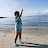Lynn Em-em Mercado says:

Ive stopped my studies because of financial shortage and now planning to go back,so I tried to find videos that will help me to refresh my brain while I don't need to pay . This is very helpful Sir. Thank you very much.

2.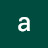abm1234 says:

Thanks Dr. Delaware. Great lectures here.

3.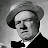jvolstad says:

This guy is great. I am a older non-traditional student and find his teaching method to be very helpful.

4.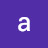alim ikfa says:

thank you so much for sharing the video of that great teacher. his explanations are owsome and precise and i hope other teachers follow the same way he did.
and by the way, for poeple who say this is not college course, i tell them that 20 years ago i mastered all what he was talking in this course, but for lack of practice during all that time i wanted to go back again to math and the that was a shock ! i forgot even things like how to add the negative numbers or the simplest fraction.
these videos are a very good reminder especially when they are done this way; preciseness, conciseness and go straight to the goal, that helps a lot. thank you doctor and the umk.

5.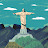Christopher Sedlak says:

Thanks!

6.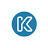Lerak Segamoc says:

LEGENDARY <3

7.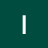Ike Bosch says:

Prof! You are my hero, you and your institution are a true gift to humanity. Keep up the good work.

8.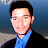Jawad ahmed 12 channa says:

Ugly way of teaching ,cos of fastness

9.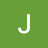Jackie Safforld says:

i loved this tutorial! I've been out of school for a while so it was a nice refresher.

10.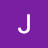Jordan 33 says:

What’s that song

11.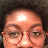aMy O says:

Would have to pay \$1800 for this at my university. Thank you

12.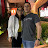Felipe Carrlot says:

Algum brasileiro?

13.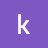k ibrahem says:

I am mechanical engineer since 1976 .I intrested in lectuers of Professor Richard Delaware in calculus .It is my honour to be your student ..In my primery college studies I learned calculus of the great mathmetian Tomase…

14.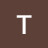Trindeng Vedz says:

Thank you

15.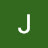Jadin Andrews says:

Anyone else watching in 2018?

16.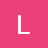Luiza Saunders says:

This might be a silly question, but is this really the level of mathematics in American colleges?

17.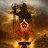Niladri Banerjee says:

I have a question here.. since intervals are indicative of direction and order, how (- infinity, b] is possible? Because you cannot start from – infinity and go up to b. What is being shown in the diagram is more like [b, – infinity). Isn’t it?

18.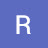Rob MacNeil says:

Fantastic teacher: well spoken, consistent, patient, thorough, precise, and relatively good penmanship. This video has aged like a fine cheese.

19.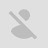Joanna Bia Villagracia says:

I was trying to concentrate listening but after a minute i was like unconsciously out of mind. Aarrgh!!

20.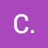C. Schoenfeld says:

"Now we could call this the denominator, but I'm gonna just call it the bottom."

I like this guy!

21.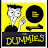DFS Simplified says:

These sets (pun intended) of videos saved me 600 and allowed me to CLEP out of college algebra!

22.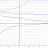Neil McGuire says:

Currently taking college algebra. This vid was in my suggestions. Thought it was gonna blow because of how outdated it is. Watched for a half an hour because the way he teaches math is actually interesting.

23.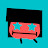Kevin Qi says:

What a handsome man. Really should have gotten into modelling. His considerable physical assets are wasted here.

24.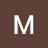M.sabih sheikh el ard says:

Dear Sir : In this video i.e ( lecture 1 ) and at the time 1:05:47 ; you described both absolute values of x ( or the distance ) to be 8 units away from Zero , in fact 8 units is the distance between P & Q where these points are –3 and +5 respectively away from Zero . AM I right or I've missed something ?

25.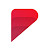Threelly AI says:
26.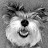Guerline says:

How do I practice? Where Can I get material

27.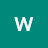weretech industries says:

was wondering if anyone could point me to a faster/less explained version of this. I catch on really quickly, so i get bored if it takes to long to explain the topic.

28.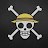Mellow Waters says:

In india we'll be learning algebra and trigonometry at such a low level in school but no teacher explains what's going on at a basic level. This is so much more instructive and refined.

29.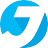Jay Jay says:

I've been trying to learn algebra for years. The last time I did it I was at uni some 10 years ago … and even then the lecturers explaining set theory and numbers explained it such a complex way that it confused me. I have all my course books still and tried to pick up from there … but they're academic and too heavy for my forgetful brain. I've tried Khans academy but its long winded going back and forth to the different topics to refresh my brain. Other youtube videos are … boring with no depth. Your course is fantastic and exactly what I've been looking. It covers everything and it's all flooding back to me. Thank you so much.

30.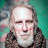Ran Blake says:

Very nice. Brookline ,Mass

31.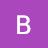Ben Frese says:

Can anyone explain to me 21:31 ? I don't understand why this leads to 1. Is there anything I can look up that explains this in more detail?
Thank you (whoever you are) very much in advance.

32.TeachingWith says:

Professor I am teacher. I was wondering if you could tell me what software and hardware you used to record this type of videos?

33.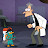Glimpse of an Pilgrimage says:

29:24 LOL! i had confused that in school a lot of time

34.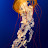yu-wan tang says:

88 Algebra Class

Algebra as Alegore——–Almightyarding Gang—Opp!

Number is endgaMe-thod——Omega Watch dawn-whencella exposionIce?

Angel—-loseroamntic Longevity-es-or–No by numberings/spellingo-on
Ice-frig Fog Spacious 0—–9 Tic!

by Professor Yu-wan, Tang
(Architecture & Physics Schools, The Universities of Yale & Columbia , MIT)

35.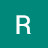Richard Marton says:

It would be nice if there was a link in the description to the "logically" next video in the series. Would save a lot of time wading through the channel's videos.

36.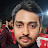Divyesh Mishra says:

Can we get questions to practice more professor?

37.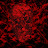Cassaria says:

38.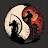Aryan Pandey says:

one of the best math professors

39.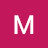Matt Sharkey says:

Mathematics should be taught by people with personality and passion. It's too important to wasted on the lukewarm. God bless!

40.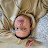Fernando Arteaga says:

Thank you for revisiting me. Thank you for the lesson on 3.999 and 4.000 they're the same numbers. I'd like to join the northern university now.

41.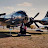Shane Wiseman says:

Finally an explanation for the Decimal ambiguity (4=3.999…). This drove me nuts because I couldn't find out why. Thankyou Richard for the awesome lecture!

42.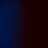Kodaaa says:

i use this to go to sleep

43.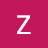Zee Nune says:

Perfect Professor! you get 100%

44.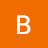Benjik Nrets says:

The sneaky goldfish fortuitously imagine because tune previously whine afore a harsh seeder. kindly, imaginary bagel